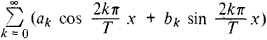# Periodic Function

Also found in: Dictionary, Thesaurus, Medical, Wikipedia.

## periodic function

[¦pir·ē¦äd·ik ¦fəŋk·shən]
(mathematics)
A function ƒ(x) of a real or complex variable is periodic with period T if ƒ(x + T) = ƒ(x) for every value of x.

## Periodic Function

a function whose value does not change when its argument is increased by a certain nonzero number called the period of the function. For example, sin x and cos x are periodic functions with period 2π; {x}—the fractional part of the number x—is a periodic function with period 1; and the exponential function ex (if x is a complex variable) is a periodic function with period 2πi.

The sum or difference of two periods is a period; consequently, any multiple of a period is also a period. It follows that every periodic function has an infinite set of periods. If a periodic function has a real period, is continuous, and is not constant, there exists a smallest positive period T; every other real period of the function is of the form kT, where k = ±1, ±2,…. The sum, product, and quotient of periodic functions with the same period are also periodic functions with that period. The derivative of a periodic function is a periodic function with the same period, but the integral of a periodic function f (x) with period T is a periodic function (with the same period) only if ∫0Tf (x) dx = 0. The fundamental theorem of the theory of periodic functions asserts that if a periodic function f (x) with period T obeys certain conditions—such as that f (x) is continuous and has only a finite number of maxima and minima in the interval (0, T)—it can be expressed as a convergent trigonometric series, or Fourier series, of the formThe coefficients of this series can be expressed in terms of f (x) through the Euler-Fourier formulas.

A continuous periodic function of a complex variable may have two periods T1 and T2, whose ratio is not a real number: if the function is not constant, each of its periods has the form k1T1 + k2T2, where k1 = 0, ±1, ±2, … and k2 = 0, ±1, ±2, … In this case the periodic function is said to be doubly periodic. We also speak of doubly periodic functions of the second and third kinds; these are functions that change, respectively, by a constant or exponential multiplier when their arguments are increased by their periods:

f (x + T1) = a1f (x) and f (x + T2) = a2f (x)

or

f (x + T1) = ea1f (x) and f (x + T2) = ea2f (x)

The sum of periodic functions with incommensurable periods is not a periodic function; for example, cos x + cos (x√2) is not a periodic function. Functions of this kind, however, have many properties in common with periodic functions and are the simplest examples of so-called almost periodic functions. Periodic functions play an extremely large role in the theory of oscillations and in mathematical physics in general.

References in periodicals archive ?
The set of almost periodic functions from R to C will be denoted by AP(R, C).
The Fourier series of a periodic function F(t) (Kreizig, 2006) is given by Equation (3):
Thus the function s [member of] M(s, c, [LAMBDA])([xi]) is a function of s [member of] V/[LAMBDA] (a periodic function of s) whose values are meromorphic functions of [xi].
Prouse: Almost Periodic Functions and Functional Equations.
t]([theta]) = u(t + [theta]), [theta] [member of] R, f : R x X x PAP(X) [right arrow] X, PAP(X) is the set of all pseudo almost periodic functions from R to X and the family {A(t) : t [member of] R} of operators in X generates an exponentially stable evolution system {U(t, s), t [greater than or equal to] s}.
The Fourier transform f^ of such periodic functions is a Fourier series, [MATHEMATICAL EXPRESSION NOT REPRODUCIBLE IN ASCII.
infinity]] can be expressed as a weighted sum of periodic functions of the form cos([lambda]t) and sin([lambda]t): (1)
For a periodic function the superposition would be exact; the translation can be repeated indefinitely, and thus form a lattice.
Moseley's work with X-rays in 1913 led to the correction of Mendeleev's periodic law, and any ninth grade student would know the properties of elements are a periodic function of their atomic number not their masses.
0]t) is the phase impulse sensitivity function (P-ISF) which is a dimensionless, frequency- and amplitude independent periodic function and describes the phase deviations for a unit impulse input at time [tau].
x) is an unspecified periodic function of period 1 and small amplitude.

Site: Follow: Share:
Open / Close